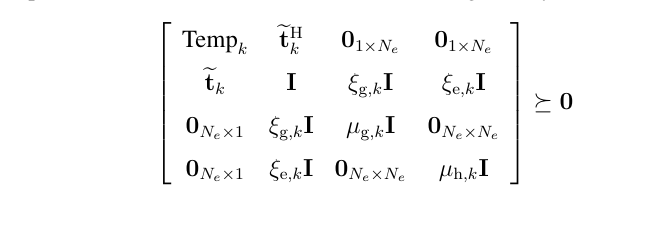# LMI constraint error

I met a LMI constraint in optimization problem as followsthe code is

[ beta1(k) - M*mu_g(k) - mu_e(k) g(:,k)’ zeros(1,E) zeros(1,E);…
g(:,k) ones(E,E) epsilon_e.*ones(E,E) epsilon_g.*ones(E,E);…
zeros(E,1) epsilon_g.*ones(E,E) mu_g(k).*ones(E,E) zeros(E,E);…
zeros(E,1) epsilon_e.*ones(E,E) zeros(E,E) mu_e(k).*ones(E,E)] >= zeros(10);

but there is an error
Disciplined convex programming error:
Invalid constraint: {complex affine} >= {constant}

b = newcnstr( evalin( ‘caller’, ‘cvx_problem’, ‘[]’ ), x, y, ‘>=’ );

[ beta1(k) - Mmu_g(k) - mu_e(k) g(:,k)’ zeros(1,E)
zeros(1,E);…
the right hand of constraint is matrix of (3
M+1)(3M+1)

Have you turned on sdp mode, by using `cvx_begin sdp` instead of `cvx_begin`?